Home Practice
For learners and parents For teachers and schools
Textbooks
Mathematics
JSS 1 JSS 2 JSS 3
Full catalogue
Pricing SupportLog in

We think you are located in Nigeria. Is this correct?

# Test yourself now

High marks in science are the key to your success and future plans. Test yourself and learn more on Siyavula Practice.

# Chapter 7: Area of plane shapes

Knowing how to calculate the area of an object has many uses, particularly when we need to know how much of a material we require to do a certain task, and want to work out how much it is going to cost. For example, when we want to paint a wall, we need to calculate the area of the wall so we can buy the right amount of paint.

Area is the size of a flat surface. (We can also call a flat surface a "plane".) We can also describe area as the amount of space inside the boundary of a plane shape such as a triangle, parallelogram, trapezium or circle. Area is measured in mm $^{2}$; cm $^{2}$; m $^{2}$ or km $^{2}$.

area Area is the amount of space taken up by a flat shape.

plane shape A plane shape is a closed shape on a flat surface, for example a triangle, parallelogram, trapezium or circle. It is also called a two-dimensional shape.

## 7.1 Area of triangles

You already know how to find the area of a rectangle. If a rectangle is drawn on a grid, as shown in the diagram below, you can simply count the small squares to find the area of the rectangle.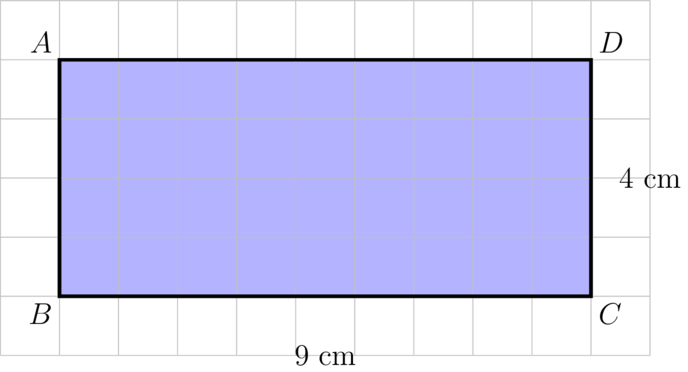Rectangle $ABCD$ consists of 9 columns of squares. Each column has 4 squares. This means there are 36 squares inside the rectangle. Each small square has dimensions of 1 cm by 1 cm, so the area of the rectangle is $36 \text{cm}^{2}$.

You can also use a formula to calculate the area of a rectangle:

\begin {align} A_{\text{rectangle}} & = \text{length} \times \text{breadth} \\ & = l \times b \\ & = \text{9 cm} \times \text{4 cm} \\ & = \text{36 cm}^{2}\\ \end{align}

We use the following formula for the area of a rectangle:

\begin{align} A_{\text{rectangle} } & = \text{length} \times \text{breadth} \\ &= l \times b \\ \end{align}

There are two other formulae that may also be used:

\begin{align} A_{\text{rectangle}} & = \text{length} \times \text{width} \\ &= l \times w \\ \end{align} \begin{align} A _{\text{rectangle}} &= \text{base} \times \text{height} \\ & = b \times h \\ \end{align}

To find the area of a triangle, we divide a rectangle into two equal parts.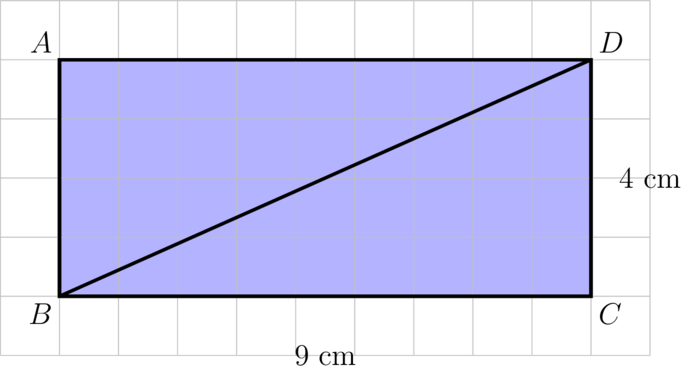$$BD$$ is a diagonal of rectangle $$ABCD$$. $$BD$$ divides the rectangle into two equal parts. The area of each triangle should then be equal to half of $$36 \text{cm}^{2}$$.

The diagram shows:

\begin{align} \text{Area } \triangle BCD &= \frac{1}{2} \text{ of area } ABCD \\ &= \frac{1}{2} \times BC \times CD \\ & = \frac{1}{2} \times 9 \times 4 \\ & = \frac{1}{2} \times 36 \\ & = \text {18 cm}^{2}\\ \end{align}

Similarly:

\begin{align} \text{Area } \triangle ABD &= \frac{1}{2} \text{ of area } ABCD \\ &= \frac{1}{2} \times AD \times BC \\ & = \frac{1}{2} \times 9 \times 4 \\ & = \frac{1}{2} \times 36 \\ & = \text {18 cm}^{2}\\ \end{align}

From the workings above, we can derive the formula for the area of a triangle:

\begin{align} \text{Area of triangle} &= \frac{1}{2} \times \text{base} \times \text{height} \end{align}

In the diagrams above, we used right-angled triangles. What will happen if we draw an acute angled triangle in the rectangle?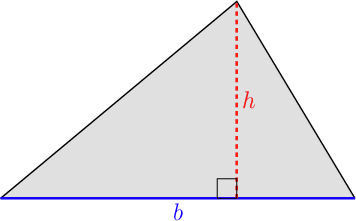We can draw a rectangle around the triangle so that:

• the length of the rectangle is equal to the base of the triangle, and
• the breadth of the rectangle is equal to the height of the triangle.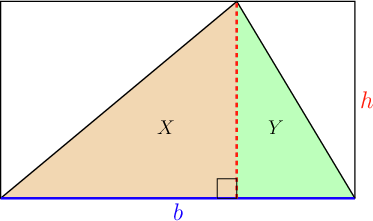Notice that our triangle is made of two right-angled triangles, $X$ and $Y$. But, the space taken up by triangle $X$ is the same as triangle $X^{\prime}$. Also, the space taken up by triangle $Y$ is the same as triangle $Y^{\prime}$.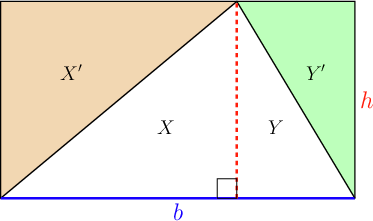So, in the diagram above, the unshaded area of our triangle ( $X$ and $Y$) is equal to the shaded area of the rectangle ( $X^{\prime}$ and $Y^{\prime}$). This means that the space taken up by our triangle is equal to half of the space taken up by a rectangle with the same base and height.

The area of the rectangle is: $A = \text{base} \times \text{height}$.

The area of the triangle is half of this, so the formula for the area of a triangle is:

The formula can be written in slightly different ways, but they all mean the same thing.

• $A_{\text{triangle}} = \frac{1}{2}bh$
• $A_{\text{triangle}} = \frac{1}{2} \times b \times h$
• $A_{\text{triangle}} = \frac{b \times h}{2}$
• $A_{\text{triangle}} = \frac{1}{2}(b \times h)$

We call the side that we choose to use in our calculations the "base" of the triangle, even if it isn't at the bottom of the triangle.

The height of a triangle must be perpendicular to the base of the triangle. The height must also cover the distance between the base and the third vertex.

perpendicular Perpendicular means that two lines meet at $$90^{\circ}$$. This is shown by drawing a little square at the angle. We write an upside down letter T to show that the base is perpendicular to the height: $\text{base} \perp \text{height }$.

• Most of the time the height of the triangle will be "inside" the triangle.• Sometimes it is necessary for the height of the triangle to be "outside" the triangle. This is so the height can be perpendicular to the base, and still touch the opposite vertex.• In a right-angled triangle, one of the sides of the triangle is the height of the triangle.### Exercise 7.1: Identify the height of a triangle

1. Consider the diagram of $\triangle PQR$.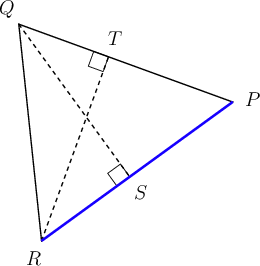If $RP$ is the base of $\triangle PQR$, what is the height that goes with that base?

The base in this question is $RP$. $QS$ is perpendicular to the base and covers the distance between the base and the third vertex, $Q$. $QS$ is therefore the height for this base.

This is easier to see when the base is horizontal: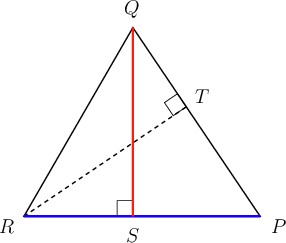$QS$ is the height if $RP$ is the base of the triangle.

2. Consider the diagram of $\triangle PQR$.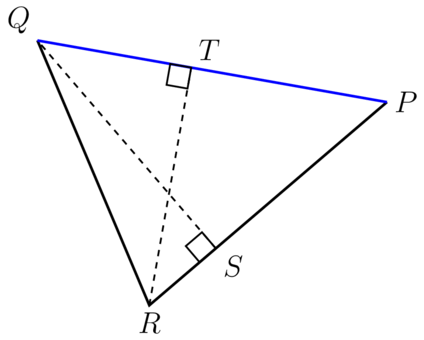If $PQ$ is the base of $\triangle PQR$, what is the height that goes with that base?

The base in this question is $PQ$. $RT$ is perpendicular to the base and covers the distance between the base and the third vertex, $R$. So $RT$ is the height.

This is easier to see when the base is horizontal: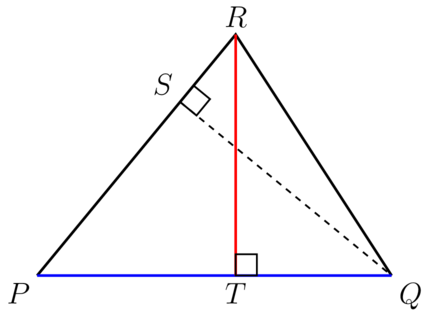$RT$ is the height if $PQ$ is the base of the triangle.

3. Consider the diagram of $\triangle PQR$.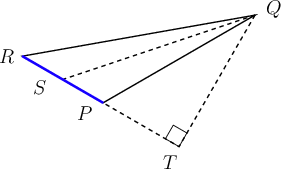If $RP$ is the base of $\triangle PQR$, what is the height that goes with that base?

The base in this question is $PR$. $QT$ is perpendicular to the base and covers the distance between the base and the third vertex, $Q$. So $QT$ is the height.

This is easier to see when the base is horizontal: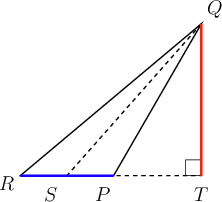$QT$ is the height if $RP$ is the base of the triangle.

When you know how to identify the base and the perpendicular height in a triangle, it is very easy to calculate the area of the triangle. Make sure you substitute the correct values into the formula and remember to use the correct units when you give the final answer.

### Worked example 7.1: Calculating area of a triangle

Find the area of the triangle below.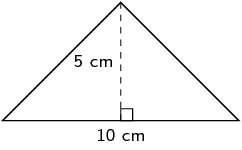1. Step 1: Write down the formula for the area of a triangle.

2. Step 2: Substitute the values for the base and the height.

\begin{align} \text{Area of } \triangle & = \frac{1}{2} \times b \times h \\ & = \frac{1}{2} \times 10 \times 5 \\ \end{align}
3. Step 3: Complete the calculation, and remember to give the correct units with the answer.

\begin{align} \text{Area of } \triangle & = \frac{1}{2} \times b \times h \\ & = \frac{1}{2} \times 10 \times 5 \\ & = \text{25 cm}^{2}\\ \end{align}

You may not be given all the information you need when you have to calculate the area of a triangle. If you have a right-angled triangle, you can use the theorem of Pythagoras.

If $\triangle ABC$ is a right-angled triangle with $\hat {B}$ equal to $90^{\circ}$, the theorem of Pythagoras states: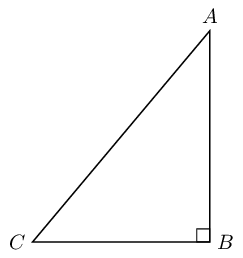You can only use the side that is opposite the right angle as the side that is equal to the sum of the squares of the other two sides. This is not true for the other sides of the triangle. In this diagram, it is $AC$ that is opposite $\hat {B}$, which is $90^{\circ}$.

### Worked example 7.2: Calculating area of a triangle using Pythagoras

Consider the triangle provided.Calculate the area of $\triangle JKL$.

1. Step 1: Identify the base and the height in the given triangle.

$JK$ is the base of the triangle and $LJ$ is the height of the triangle.

$LJ$ is given as 8 cm, but the length of $JK$ is unknown.

2. Step 2: Calculate the length of $JK$, using the theorem of Pythagoras.

The side opposite the right angle is the side marked 10 cm, so:

\begin{align} JK^{2} + 8^{2} & = 10^{2} \quad (\text{Pythagoras}) \\ JK^{2} + 64 & = 100 \\ JK^{2} + 64 - 64 & = 100 - 64 \\ JK^{2} & = 36 \\ JK & = \sqrt{36} \\ & = \text{6 cm} \\ \end{align}
3. Step 3: Write down the formula for the area of a triangle.

4. Step 4: Substitute the values for the base and the height.

\begin{align} \text{Area of } \triangle & = \frac{1}{2} \times b \times h \\ & = \frac{1}{2} \times 6 \times 8 \\ \end{align}
5. Step 5: Complete the calculation, and remember to give the correct units with the answer.

\begin{align} \text{Area of } \triangle & = \frac{1}{2} \times b \times h \\ & = \frac{1}{2} \times 6 \times 8 \\ & = \text{24 cm}^{2}\\ \end{align}

If two sides of a triangle are perpendicular to each other, the height can be either of these sides of the triangle. This will only happen in right-angled triangles.

It does not matter which one of these sides is the "base" or the "perpendicular height", because when you multiply one by the other, you get the same answer.

### Exercise 7.2: Calculate area of a triangle

1. Calculate the area of $\triangle XZY$ provided.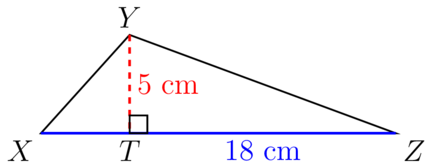\begin{align} \text{Area } \triangle XYZ &= \frac{1}{2} \times b \times h \\ & = \frac{1}{2} \times 18 \times 5 \\ & = \text{45}\ {\text{cm}^{2}} \\ \end{align}
2. Calculate the area of $\triangle XYZ$.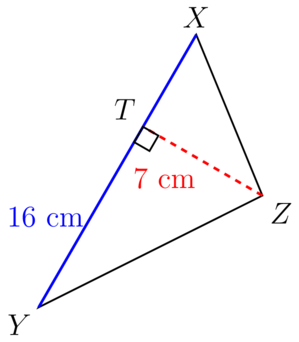\begin{align} A_{\text{triangle}} & = \frac{1}{2} \times b \times h \\ & = \frac{1}{2} \times 16 \times 7 \\ & = \text{56}\ {\text{cm}^{2}} \\ \end{align}
3. Calculate the area of the given triangle, $\triangle KJL$.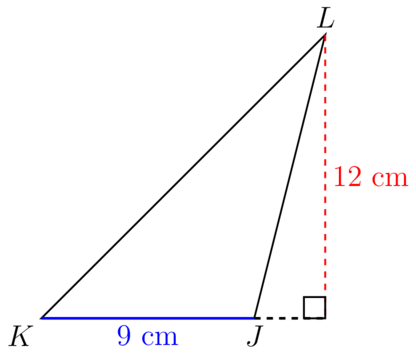\begin{align} A_{\text{triangle}} & = \frac{1}{2} \times b \times h \\ &= \frac{1}{2} \times 9 \times 12 \\ & = \text{54 cm}^{2} \\ \end{align}
4. Calculate the area of the triangle provided.We are going to treat $DE$ as the base and $EF$ as the height.

\begin{align} A_{\text{triangle}} & = \frac{1}{2} \times b \times h \\ & = \frac{1}{2} \times 12 \times 5 \\ & = \text{30 m}^{2} \\ \end{align}
5. Consider the diagram of $\triangle DFE$ provided.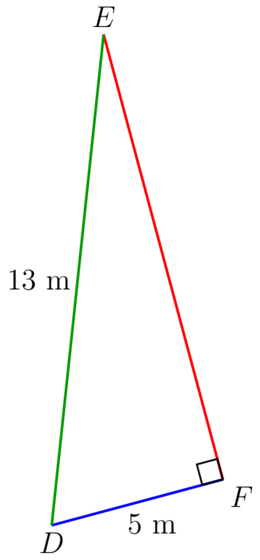Calculate the area of $\triangle DFE$.

Calculate the length of $EF$ first.

\begin{align} EF^{2} + DF^{2} &=ED^{2} \quad (\text{Pythagoras})\\ EF^{2} + 5^{2} & = 13^{2}\\ EF^{2} + 25 & = 169\\ EF^{2} + 25 - 25 & = 169 - 25\\ EF^{2} & = 144\\ \therefore EF & = \sqrt {144}\\ EF & = \text{12 m}\\ \end{align}

Now calculate the area of $\triangle DFE$.

\begin{align} A_{\text{triangle}} & = \frac{1}{2} \times b \times h \\ & = \frac{1}{2} \times 5 \times 12 \\ & = \text{30}\ {\text{m}^{2}} \\ \end{align}
6. Consider the diagram of $\triangle JLK$ below.

• $JL = \text{39}\ \text{m}$
• $LK = \text{25}\ \text{m}$
• $JK = \text{40}\ \text{m}$
• $KM = \text{24}\ \text{m}$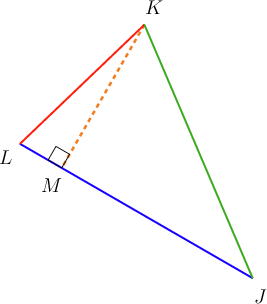Calculate the area of $\triangle JLK$.

$KM$ is given as the perpendicular height of the triangle, so we must use $JL$ as the base.

\begin{align} A_{\text{triangle}} & = \frac{1}{2} \times b \times h \\ & = \frac{1}{2} \times 39 \times 24 \\ & = \text{468 m}^{2} \\ \end{align}
7. Consider the diagram of $\triangle ZYX$ provided.

• $ZY = \text{21}\ \text{cm}$
• $YX = \text{13}\ \text{cm}$
• $ZX = \text{20}\ \text{cm}$
• $XT = \text{12}\ \text{cm}$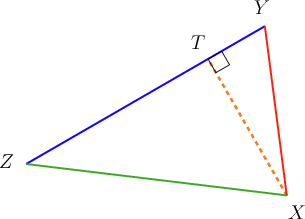Calculate the area of $\triangle ZYX$.

$XT$ is given as the perpendicular height of the triangle, so we must use $ZY$ as the base.

\begin{align} A_{\text{triangle}} & = \frac{1}{2} \times b \times h \\ & = \frac{1}{2} \times 21 \times 12 \\ & = \text{126 cm}^{2} \\ \end{align}
8. Find the area of the given triangle.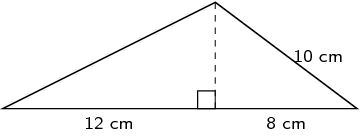The base of the triangle = $\text{12 cm} + \text{8 cm} = \text{20 cm}$.

We first need to work out the height ( $h$) of the triangle, using the theorem of Pythagoras. The line marked 10 cm is opposite the right angle.

\begin{align} h^{2} + 8^{2} & = 10^{2} \quad (\text{Pythagoras})\\ h^{2} + 64 & = 100 \\ h^{2} + 64 - 64& = 100 - 64\\ h^{2} & = 36\\ \therefore h & = \sqrt{36} \\ h & = \text{6 cm} \\ \end{align}

Now we can calculate the area of the triangle:

\begin{align} A_{\text{triangle}} & = \frac{1}{2} \times b \times h \\ & = \frac{1}{2} \times 6 \times 20 \\ & = \text{60 cm}^{2} \\ \end{align}

We can use our knowledge about the area of a triangle to derive the formulas for the area of quadrilaterals.

quadrilateral A quadrilateral is a flat shape with four sides and four angles.

We will focus on two quadrilaterals in this chapter, namely a parallelogram and a trapezium.

### Area of a parallelogram

A parallelogram is a quadrilateral with two pairs of opposite sides that are equal and parallel.

The diagram below shows that a parallelogram can be divided into two triangles.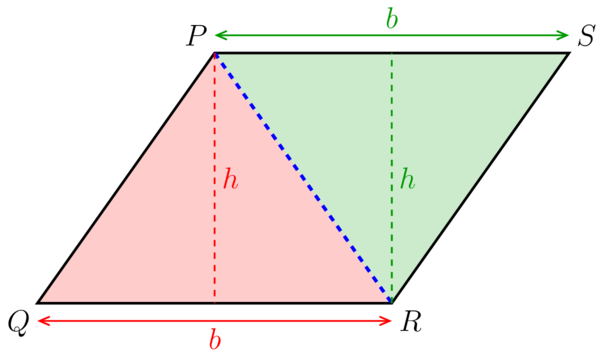We can see that the area of parallelogram $PQRS$ is equal to the sum of the area of $\triangle PQR$ and the area of $\triangle PSR$.

Remember the following:

• The opposite sides of a parallelogram are equal, so $PS$ = $QR$. This means that the base of $\triangle PQR$ is exactly the same as the base of $\triangle PSR$.
• The opposite sides of a parallelogram are parallel, so the distance between $PS$ and $QR$ stays the same. This means that the height of $\triangle PQR$ is exactly the same as the height of $\triangle PSR$.
\begin{align} \text{Area parallelogram PQRS} & = \text{Area } \triangle PQR + \text{area } \triangle PSR\\ & = \frac{1}{2} \times b \times h + \frac{1}{2} \times b \times h \\ & = 1 \times b \times h \\ & = b \times h \\ \end{align}

The formula for the area of a parallelogram is therefore:

It is very important to remember that the base ( $b$) and the height ( $h$) are perpendicular to each other.### Worked example 7.3: Calculating area of a parallelogram

Calculate the area of the parallelogram given below.1. Step 1: Write down the formula to calculate the area of a parallelogram.

$A_{\text{parallelogram}}$ = $\text{base} \times \text{height}$

2. Step 2: Identify the base and the height. Substitute these values into the formula.

\begin{align} A_{\text{parallelogram}} &= b \times h\\ & = 6 \times 3 \\ \end{align}
3. Step 3: Complete the calculation, giving the correct units with the answer.

\begin{align} A_{\text{parallelogram}} &= b \times h\\ & = 6 \times 3 \\ & = \text{18 cm}^{2} \\ \end{align}

### Exercise 7.3: Calculate area of a parallelogram

1. Write down the formula to calculate the area of a parallelogram. Explain the meaning of each variable in the formula.

Formula:

$A_{\text{parallelogram}}$ = $b \times h$

Remember that a variable is a symbol or a letter that represents an unknown number.

Variables:

• $b$ represents the base of the parallelogram.
• $h$ represents the height of the parallelogram.

The height must be perpendicular to the base.

2. Calculate the area of the three parallelograms given below.\begin{align} A_{\text{parallelogram}} &= b \times h\\ & = 90 \times 30 \\ & = \text{2,700 mm}^{2} \\ \end{align}\begin{align} A_{\text{parallelogram}} &= b \times h\\ & = 4 \times 1.5 \\ & = \text{6 m}^{2} \\ \end{align}\begin{align} A_{\text{parallelogram}} &= b \times h\\ & = 12 \times 7 \\ & = \text{84 cm}^{2} \\ \end{align}
3. Find the area of the following parallelogram.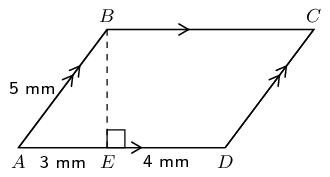$BE$ is the height of the parallelogram.

Use the theorem of Pythagoras to find the length of $BE$. Notice that $AB$ is the side opposite the right angle.

\begin{align} BE^{2} + AE^{2} & = AB^{2} \quad (\text{Pythagoras})\\ BE^{2} + 3^{2} & = 5^{2}\\ BE^{2} + 9 & = 25 \\ BE^{2} + 9 - 9 & = 25 - 9 \\ BE^{2} & = 16 \\ \therefore BE & = \sqrt {16} \\ & = \text{4 mm} \\ \end{align}

Find the area using using the correct formula.

\begin{align} A_{\text{parallelogram}} & = b \times h \\ & = AD \times BE\\ & = (3 + 4 ) \times 4 \\ & = 7 \times 4\\ & = \text{28 mm}^{2}\\ \end{align}
4. Consider the polygon given below. Identify the polygon (giving a reason for your answer), and determine the area of the polygon.$PQRS$ is a parallelogram, because it has two pairs of parallel sides.

First work out the height of the parallelogram, using the theorem of Pythagoras in $\triangle RST$.

\begin{align} RT^{2} + TS^{2} & = RS^{2} \quad (\text{Pythagoras})\\ RT^{2} + 3^{2} & = 5^{2}\\ RT^{2} + 9 & = 25 \\ RT^{2} + 9 - 9 & = 25 - 9 \\ RT^{2} & = 16 \\ \therefore RT & = \sqrt {16} \\ & = \text{4 mm} \\ \end{align}

Now calculate the area.

\begin{align} A_{\text{parallelogram}} & = b \times h \\ & = PS \times TR\\ & = (3 + 7 ) \times 4 \\ & = 10 \times 4 \\ & = \text{40 cm}^{2}\\ \end{align}

### Area of a trapezium

A trapezium is a quadrilateral with one pair of opposite sides that are parallel.

The diagram below shows that a trapezium can be divided into two triangles.We can see that the area of trapezium $PQRS$ is equal to the sum of the area of $\triangle PQR$ and the area of $\triangle PSR$.

Remember the following:

• One pair of opposite sides of a trapezium are parallel, so the distance between $PS$ and $QR$ stays the same. This means that the height of $\triangle PQR$ is exactly the same as the height of $\triangle PSR$.
\begin{align} \text{Area trapezium PQRS} & = \text{area } \triangle PQR + \text{area } \triangle PSR\\ & = \frac{1}{2} \times QR \times h + \frac{1}{2} \times PS \times h \\ &= \frac{1}{2} \times h \left (QR + PS \right)\\ \end{align}

We can use the variables $a$ and $b$ for the lengths of the two parallel sides, and then we write the formula as follows:

We know that $\frac{1}{2} \times h = \frac{h}{2}$, so we can write the formula as follows:

The two parallel sides of the trapezium ( $a$ and $b$) must be perpendicular to the height ( $h$) of the trapezium.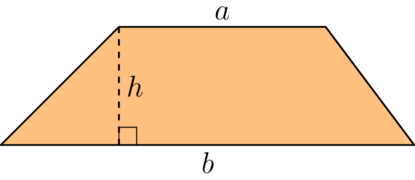### Worked example 7.4: Calculating area of a trapezium

Determine the area of the trapezium given below.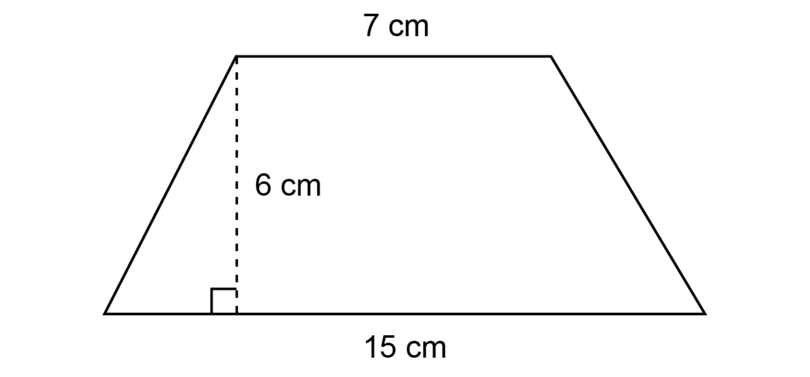1. Step 1: Write down the correct formula.

2. Step 2: Substitute the correct values into the formula.

\begin{align} A_{\text{trapezium}} &= \frac{h}{2}\left( a + b \right) \\ & = \frac{6 }{2}\left( 7 + 15 \right)\\ \end{align}
3. Step 3: Complete the calculation, giving the correct units with the answer.

\begin{align} A_{\text{trapezium}} &= \frac{h}{2}\left( a + b \right) \\ & = \frac{6 }{2}\left( 7 + 15 \right)\\ & = 3 \times \left(22 \right)\\ & = \text{66 cm}^{2}\\ \end{align}

### Exercise 7.4: Calculate area of a trapezium

1. For each of the shapes shown below, identify the correct formula for the area of the shape.

Shape A Shape B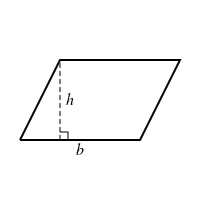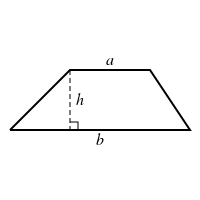Choice Area formula
1 $A = b^{2}$
2 $A = bh$
3 $A = \frac{h}{2}\left( a + b \right)$
4 $A = \pi r^{2}$
5 $A = \frac{1}{2}bh$
• Shape A: $A = bh$ (Choice 2)
• Shape B: $A = \frac{h}{2}\left( a + b \right)$ (Choice 3)
2. Determine the areas of the three trapeziums given below.\begin{align} A_{\text{trapezium}} &= \frac{h}{2}\left( a + b \right) \\ & = \frac{8 }{2}\left( 14 + 7 \right)\\ & = 4 \times \left(21 \right)\\ & = \text{84 m}^{2}\\ \end{align}\begin{align} A_{\text{trapezium}} &= \frac{h}{2}\left( a + b \right) \\ & = \frac{40 }{2}\left( 120 + 180 \right)\\ & = 20 \times \left(300 \right)\\ & = \text{6,000 mm}^{2}\\ \end{align}\begin{align} A_{\text{trapezium}} &= \frac{h}{2}\left( a + b \right) \\ & = \frac{8 }{2}\left( 9.6 + 5.4 \right)\\ & = 4 \times \left(15 \right)\\ & = \text{60 m}^{2}\\ \end{align}
3. Identify the polygon provided, giving a reason for your answer, and determine the area of the polygon.Identify the polygon (giving a reason for the answer) and determine the area of the polygon.

$ABDE$ is a trapezium, because the polygon has one pair of parallel sides.

First work out the height of the trapezium, using the theorem of Pythagoras in $\triangle ABC$.

\begin{align} AC^{2} + BC^{2} & = AB^{2} \quad (\text{Pythagoras})\\ AC^{2} + 9^{2} & = 15^{2}\\ AC^{2} + 81 & = 225 \\ AC^{2} + 81 - 81 & = 225 - 81 \\ AC^{2} & = 144 \\ \therefore AC & = \sqrt {144} \\ & = \text{12 cm} \\ \end{align}

Now calculate the area.

\begin{align} A_{\text{trapezium}} &= \frac{h}{2}\left( a + b \right) \\ & = \frac{12 }{2}\left( 16 + 9 + 21 \right)\\ & = 6 \times \left(46 \right)\\ & = \text{276 cm}^{2}\\ \end{align}
4. Find an expression for the area of the figure below in terms of $h$ and $z$.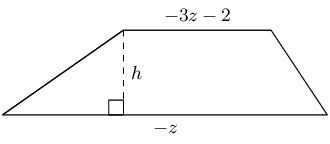\begin{array}{ll} A_{\text{trapezium}} &= \frac{h}{2}\left( a + b \right) \\ & = \frac{h}{2}\left[ (-3z - 2) + (-z) \right]\\ & = \frac{h}{2}\left( -4z - 2 \right)\\ & = (-2hz - h) \text{units}^{2}\\ \end{array}

## 7.3 Area of circles and sectors

In this section you will calculate the area of circles and sectors of circles. You have learned about the different parts of a circle in previous years:

• A diameter of a circle is a line segment that joins two points on the circumference of the circle and passes through the centre of the circle. The diameter of a circle is double the length of the radius of the same circle.
• A radius of a circle is a line segment that joins the centre of the circle to a point on the circumference. The radius of a circle is half the length of the diameter of the same circle.
• A sector of a circle is the area enclosed between two radii and the circumference of the circle.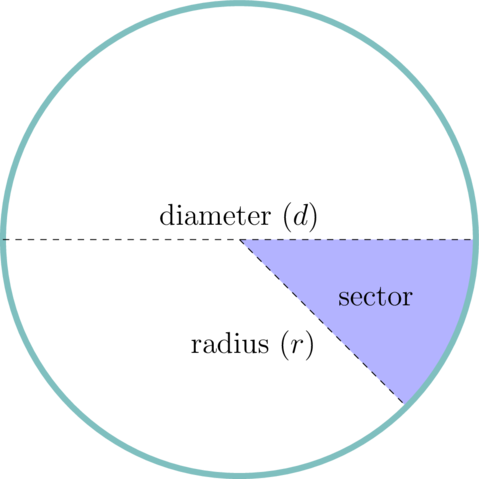### Area of a whole circle

The circumference of a circle is the curve forming the outer edge of the circle, and it is not easy to measure its length. But mathematicians have worked out that there is a special relationship between the diameter of a circle and its circumference.

circumference The circumference is the perimeter of a circle.

For any circle, no matter how big or small, if you divide the circumference ( $C$) by the diameter ( $d$), you will get a number close to 3. The more accurately you measure the circumference and diameter, the closer you will get to this special number:

This number is so special that it is given its own name, "pi". We use the symbol $\pi$ to represent pi. $\pi$ is an irrational number, so its decimal places go on forever without a pattern. (If you have a scientific calculator, a good approximation of this number is stored in it $-$ look for the $\pi$ button.)

$\pi$ $\pi$ (pi) is the ratio of the circumference of a circle to its diameter. The values we can use for $\pi$ in calculations are 3.14 and $\frac{22}{7}$.

If we multiply both sides of this equation by $d$, we get the formula for circumference of a circle:

The diameter of a circle is always double the length of the radius, so $d = 2r$. We can also write the formula as:

We will now use this last formula to help us find the area of a circle.

The area of a circle is the amount of flat space taken up by the circle. It is measured in unit squares, like mm $^{2}$; cm $^{2}$; m $^{2}$ or km $^{2}$.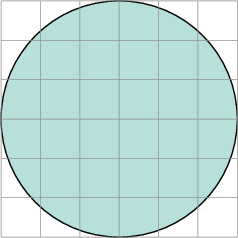It is not possible to count the unit squares taken up by a circle accurately.

Here is a fun activity for you to do to find out what the formula for the area of a circle is.

Draw a big circle and show the circumference with a thick line in a different colour. Divide the circle into at least eight sectors.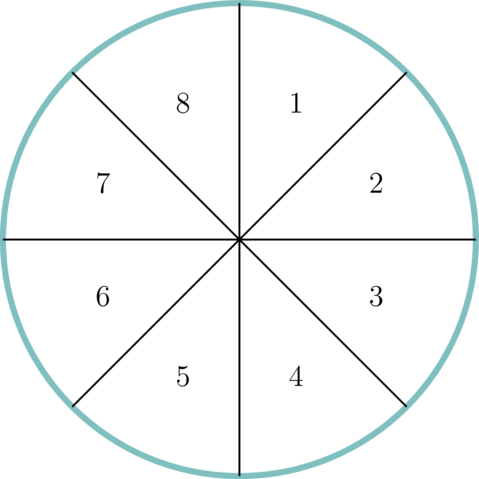Cut out the sectors and arrange them as shown below.

You have to cut one of the sectors into two halves to place first and last.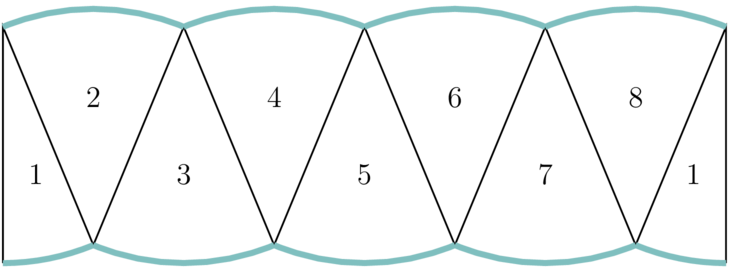The shape that you have arranged is almost a rectangle. (If you cut the circle into smaller sectors, the shape will look even more like a rectangle.)

You know that the formula for the area of a rectangle is:

Now that our circle is almost in the shape of a rectangle, we can use this formula to find the formula for the area of the circle.

Take a good look at the rectangle.

• Length of the rectangle: The thick lines that formed the circumference of the circle are now the two lengths of the rectangle. So, one length of the rectangle is equal to half of the circumference of the circle.

• Breadth of the rectangle: The breadth of the rectangle is equal to the radius of the circle.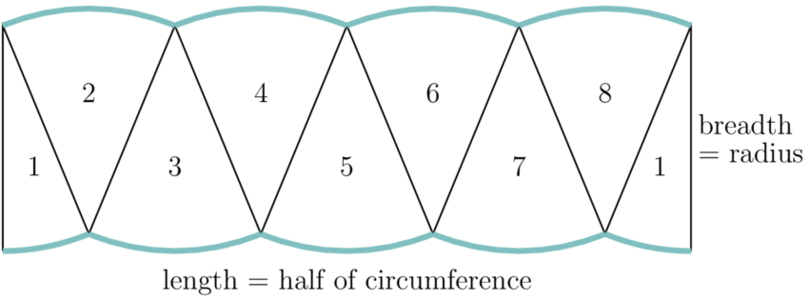\begin{align} A_{\text{circle}} & = \text{length} \times \text{breadth} \text{ (of rectangle formed by circle sectors)} \\ &= \frac{1}{2} \times \text{circumference} \times \text{radius} \\ &= \frac{1}{2} \times 2 \pi r \times r \\ &= \pi r \times r \\ &= \pi \times r^{2} \\ \end{align}

Remember that the variable $r$ stands for the radius of the circle.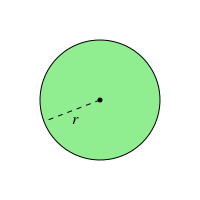Formula to calculate the area of a circle:

To help you to remember the difference between the formulas for circumference and area of a circle:

• The formula for circumference of a circle is $C = \pi d$ or $C = 2\pi r$. Circumference is measured in units of length, so nothing is squared in the circumference formula.
• The formula for area of a circle is $A = \pi r^{2}$. Area is measured in square units, so there is a square in the area formula $-$ $r^2$.

### Worked example 7.5: Calculating area of a whole circle

A circle has a diameter of 6 cm. What is its area in cm $^{2}$?

Use $\pi = 3.14$ and round your answer to two decimal places if necessary.

1. Step 1: Write down the formula for the area of a circle.

\begin{align} A_{\text{circle}} & = \pi r^{2} \\ \end{align}
2. Step 2: Substitute the correct values into the formula.

The diameter of the circle is 6 cm, so the radius of the circle is 3 cm.

3. Step 3: Complete the calculation, giving the correct units with the answer.

\begin{align} A_{\text{circle}} & = \pi r^{2} \\ & = 3.14 \times 3^{2} \\ & = 3.14 \times 9 \\ & = \text{26.26 cm}^{2} \\ \end{align}

### Exercise 7.5: Calculate area of a whole circle

Use $\pi = 3.14$ and round your answers to two decimal places where necessary.

1. For each of the shapes shown below, identify the correct formula for the area of the shape.

Shape A Shape B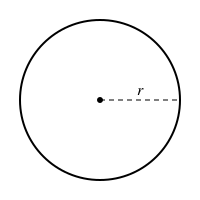Choice Area formula
1 $A = 2 \pi r$
2 $A = bh$
3 $A = \frac{h}{2}\left( a + b \right)$
4 $A = \frac{bh}{2}$
5 $A = \pi r^{2}$
• Shape A parallelogram: $A = bh$ (Choice 2)
• Shape B circle: $A = \pi r^{2}$ (Choice 5)

Other choices:

• Choice 1: Circumference of circle
• Choice 3: Area of trapezium
• Choice 4: Area of triangle
2. Determine the area of the circle given below.The radius is half the diameter, therefore the radius is 11 cm.

\begin{align} A_{\text{circle}} & = \pi r^{2} \\ & = 3.14 \times 11^{2} \\ & = 3.14 \times 121 \\ & = \text{379.94 cm}^{2} \\ \end{align}
3. The circle below has a radius of 2 km.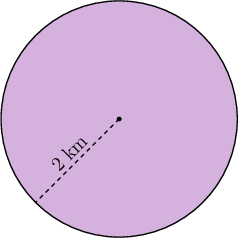What is the area of the circle?

\begin{align} A_{\text{circle}} & = \pi r^{2} \\ & = 3.14 \times 2^{2} \\ & = 3.14 \times 4 \\ & = \text{12.56 km}^{2} \\ \end{align}
4. The circle below has a radius of 10 cm.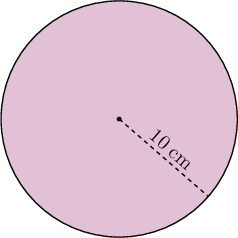What is the area of the circle?

\begin{align} A_{\text{circle}} & = \pi r^{2} \\ & = 3.14 \times 10^{2} \\ & = 3.14 \times 100 \\ & = {\text{314 cm}}^{2} \\ \end{align}
5. A circle has a radius of 1.2 m. What is its area in cm $^{2}$?

We know that 1 m = 100 cm, so we need to multiply the radius given in metres by 100.

\begin{align} r & = \text{1.2 m} \\ & = 1.2 \times \text{100 cm} \\ & = \text{120 cm} \\ \end{align}

Now we can calculate the area of the circle in cm $^{2}$.

\begin{align} A_{\text{circle}} & = \pi r^{2} \\ & = 3.14 \times 120^{2} \\ & = 3.14 \times 14,400 \\ & = \text{45,216 cm}^{2} \\ \end{align}
6. A circle has a diameter of 140 mm. What is its area in cm $^{2}$?

Start by finding the radius of the circle in centimetres. 10 mm = 1 cm

\begin{align} r & = d \div 2 \\ & = \text{140 mm} \div 2 \\ & = \text{70 mm}\\ & = \text{7 cm}\\ \end{align}

Now we can calculate the area of the circle in cm $^{2}$.

\begin{align} A_{\text{circle}} & = \pi r^{2} \\ & = 3.14 \times 7^{2} \\ & = 3.14 \times 49 \\ & = \text{153.86 cm}^{2} \\ \end{align}

### Areas of semi-circles and quarter-circles

Sometimes we do not work with a whole circle.

A semi-circle is a half of a whole circle.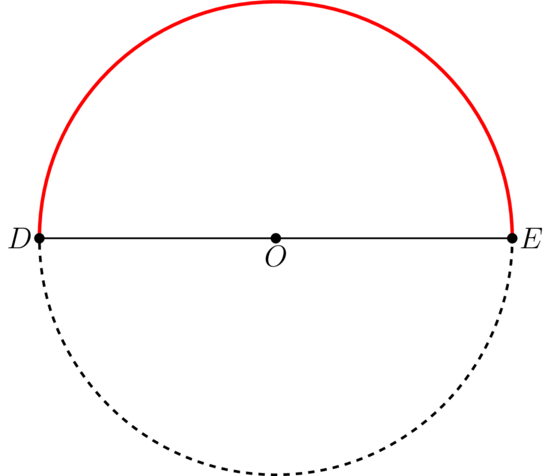The area of a semi-circle is $\frac{1}{2}$ of the area of the whole circle.

So, when you are asked to determine the area of a semi-circle, you can calculate the area of the whole circle and multiply the answer by one-half (or divide it by 2).

A quarter-circle is a quarter of a whole circle.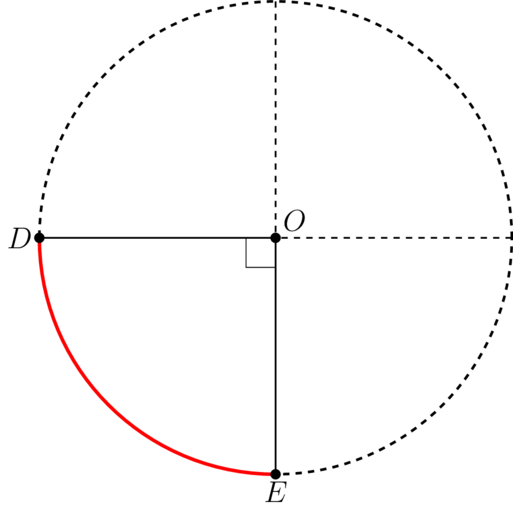The area of a quarter-circle is $\frac{1}{4}$ of the area of the whole circle.

So, when you are asked to determine the area of a quarter-circle, you can calculate the area of the whole circle and multiply the answer by one quarter (or divide it by 4).

### Worked example 7.6: Calculating area of a semi-circle

The diagram below shows a semi-circle with a diameter of 8 m.Determine the area of the semi-circle.

1. Step 1: Calculate the area of a whole circle.

The diameter of the circle is 8 m, so the radius is 4 m.

\begin{align} A_{\text{whole circle}} & = \pi r^{2} \\ & = 3.14 \times 4^{2} \\ & = 3.14 \times 16 \\ & = \text{50.24 m}^{2} \\ \end{align}
2. Step 2: Find the area of the semi-circle.

\begin{align} A_{\text{semi-circle}} & = A_{\text{whole circle}} \times \frac{1}{2} \\ & = 50.24 \times \frac{1}{2} \\ & = \text{25.12 m}^{2}\\ \end{align}

### Worked example 7.7: Calculating area of a quarter-circle

In the diagram below, $O$ is the centre of the quarter-circle with a radius of 20 cm.Determine the area of the quarter-circle.

1. Step 1: Calculate the area of a whole circle.

\begin{align} A_{\text{whole circle}} & = \pi r^{2} \\ & = 3.14 \times 20^{2} \\ & = 3.14 \times 400 \\ & = \text{1,256 cm}^{2} \\ \end{align}
2. Step 2: Find the area of the quarter-circle.

\begin{align} A_\text{quarter-circle} & = A_{\text{whole circle}} \times \frac{1}{4} \\ & = 1,256 \times \frac{1}{4} \\ & = \text{314 cm}^{2}\\ \end{align}

### Exercise 7.6: Calculate area of semi-circles and quarter-circles

1. The diagram below shows a semi-circle with a diameter of 42 cm.Determine the area of the semi-circle.

\begin{align} r &= d \div 2 \\ & = 42 \div 2 \\ & = \text{21 cm} \\ \end{align}

Area of whole circle:

\begin{align} A_{\text{whole circle}} & = \pi r^{2} \\ & = 3.14 \times 21^{2} \\ & = 3.14 \times 441 \\ & = \text{1,384.74 cm}^{2}\\ \end{align}

Area of semi-circle:

\begin{align} A_{\text{semi-circle}} & = A_{\text{whole circle}} \times \frac{1}{2} \\ & = 1,384.74 \times \frac{1}{2} \\ & = \text{692.37 cm}^{2}\\ \end{align}
2. In the diagram below, $O$ is the centre of the quarter-circle with a radius of 15 mm.Determine the area of the quarter-circle.

Area of whole circle:

\begin{align} A_{\text{whole circle}} & = \pi r^{2} \\ & = 3.14 \times 15^{2} \\ & = 3.14 \times 225 \\ & = \text{706.5 mm}^{2}\\ \end{align}

Area of quarter-circle:

\begin{align} A_{\text{quarter-circle}} & = A_{\text{whole circle}} \times {1}{4} \\ & = 706.5 \times \frac{1}{4} \\ & = \text{176.625 cm}^{2}\\ & \approx \text{176.63 cm}^{2}\\ \end{align}
3. Two shapes are given:

• Shape A is a semi-circle with radius of 7 cm.
• Shape B is a quarter-circle with radius of 10 cm.

Which of the two shapes, do you think, has the greater area?

Do the necessary calculations to see if your answer was correct or not.

Area of whole circle A:

\begin{align} A_{\text{whole circle}} & = \pi r^{2} \\ & = 3.14 \times 7^{2} \\ & = 3.14 \times 49 \\ & = \text{153.86 cm}^{2}\\ \end{align}

Area of semi-circle A:

\begin{align} A_{\text{semi-circle}} & = A_{\text{whole circle}} \times \frac{1}{2} \\ & = 153.86 \times \frac{1}{2} \\ & = \text{76.93 cm}^{2}\\ \end{align}

Area of whole circle B:

\begin{align} A_{\text{whole circle}} & = \pi r^{2} \\ & = 3.14 \times 10^{2} \\ & = 3.14 \times 100 \\ & = \text{314 cm}^{2}\\ \end{align}

Area of quarter-circle B:

\begin{align} A_{\text{quarter-circle}} & = A_{\text{whole circle}} \times \frac{1}{4} \\ & = 314 \times \frac{1}{4} \\ & = \text{78.5 cm}^{2}\\ \end{align}

Conclusion: The area of B is greater than the area of A.

So, a quarter-circle with radius 10 cm has a greater area than a semi-circle with radius 7 cm.

### Area of other sectors of circles

A sector of a circle is the area enclosed between two radii and the circumference of the circle.

• If we divide a whole circle into eight equal sectors, and we shade any four of those sectors, we have shaded an area that is half of the whole circle.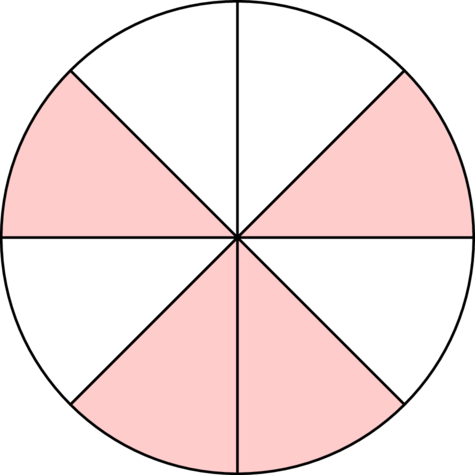• If we divide a whole circle into twelve equal sectors, and we shade any four of those sectors, we have shaded an area that is a quarter of the whole circle.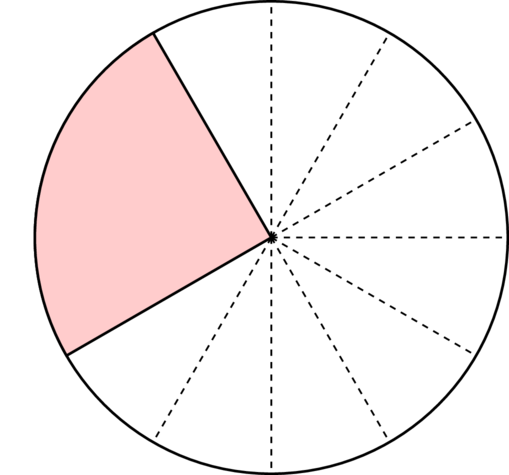We can make a sector any size, so that the sector is any fraction of the whole circle.

In the diagram below, $O$ is the centre of the circle.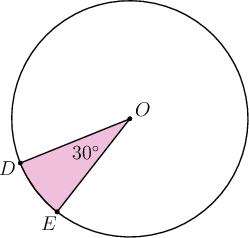A sector with an angle of $30^{\circ}$ is shaded in the circle.

You know that there are $360^{\circ}$ in a revolution. Therefore, the angles around point $O$ is equal to $360^{\circ}$.

We can calculate what fraction of the area of the circle is shaded:

So, the shaded region is $\frac{1}{12}$ of the area of the whole circle.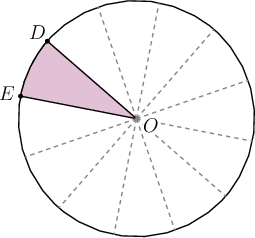### Worked example 7.8: Calculating area of a sector of a circle

Calculate the area of the shaded sector of the circle below. The radius of the circle is 24 cm.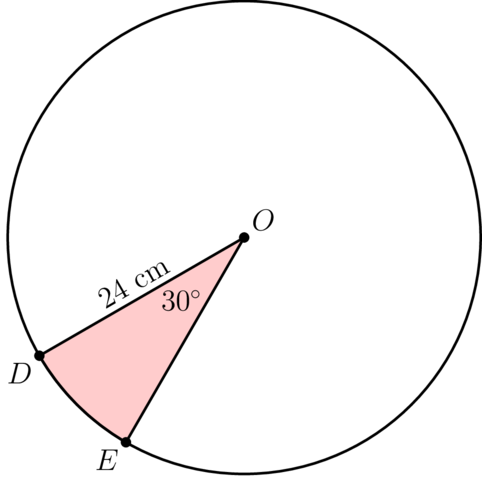1. Step 1: Work out what fraction of the circle is shaded.

Fraction shaded = $\frac{30^{\circ}}{360^{\circ} }$ = $\frac{1}{12}$

2. Step 2: Calculate the area of the whole circle.

\begin{align} A_{\text{whole circle}} & = \pi r^{2} \\ & = 3.14 \times 24^{2} \\ & = 3.14 \times 576 \\ & = \text{1,808.64 cm}^{2}\\ \end{align}
3. Step 3: Calculate the area of the shaded sector.

\begin{align} A_{\text{sector}} & = A_{\text{whole circle}} \times \frac{1}{12}\\ & = 1,808.64 \times \frac{1}{12} \\ & = \text{150.72 cm}^{2}\\ \end{align}

You will often be given the instruction to round your answer off to two decimal places. It is more accurate to round off only when you give the final answer, and not in any of the earlier steps. When you round off, you can use the symbol $\approx$, which means "approximately equal to", in your last step.

### Exercise 7.7: Calculate area of sector of a circle

1. In the diagram below, $O$ is the centre of the circle. The radius of the circle is 4.8 cm.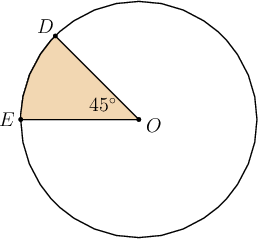Calculate the area of the shaded sector of the circle.

Calculate what fraction of the area of the circle is shaded:

So, the shaded region is $\frac{1}{8}$ of the area of the whole circle.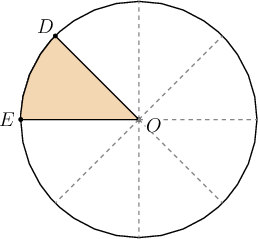Area of whole circle:

\begin{align} A_{\text{whole circle}} & = \pi r^{2} \\ & = 3.14 \times 4.8^{2} \\ & = 3.14 \times 23.04 \\ & = \text{72.3456 cm}^{2}\\ \end{align}

\begin{align} A_{\text{sector}} & = A_{\text{whole circle}} \times \frac{1}{8} \\ & = 72.3456 \times \frac{1}{8} \\ & = \text{9.0432 cm}^{2}\\ & \approx \text{9.04 m}^{2}\\ \end{align}
2. In the diagram below, $O$ is the centre of the circle. The diameter of the circle is 144 mm.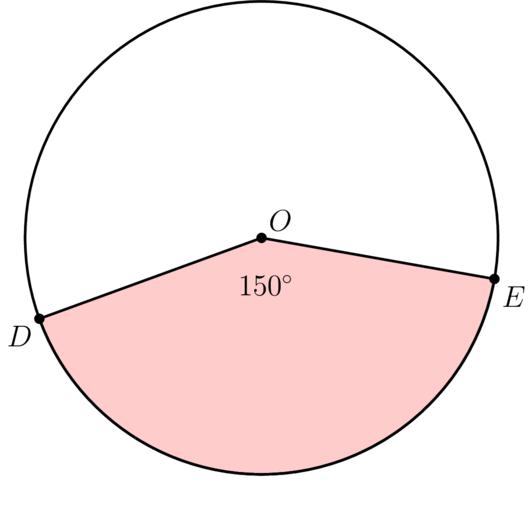Calculate the area of the shaded sector of the circle.

Calculate what fraction of the area of the circle is shaded:

So, the shaded region is $\frac{5}{12}$ of the area of the whole circle.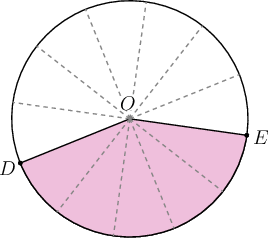Area of whole circle:

Diameter = 144 mm, so radius = 72 mm

\begin{align} A_{\text{whole circle}} & = \pi r^{2} \\ & = 3.14 \times 72^{2} \\ & = 3.14 \times 5,184 \\ & = \text{16,277.76 mm}^{2}\\ \end{align}

\begin{align} A_{\text{sector}} & = A_{\text{whole circle}} \times \frac{5}{12}\\ & = 16,277.76 \times \frac{5}{12} \\ & = \text{6,782.4 mm}^{2}\\ \end{align}
3. In the diagram below, $O$ is the centre of the circle. The radius of the circle is 60 mm. Calculate the area of the shaded sector of the circle.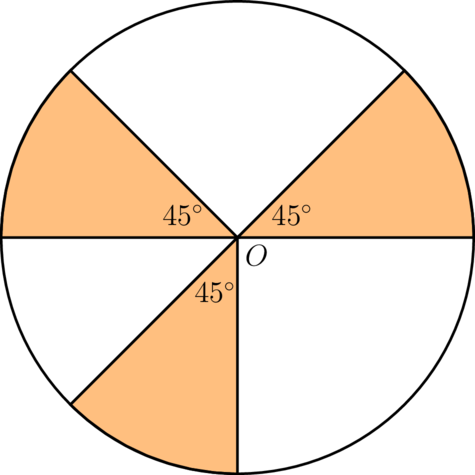Calculate what fraction of the area of the circle is shaded:

\begin{align} \frac{3 \times 45^{\circ}}{360^{\circ}} &= \frac{135^{\circ}}{360^{\circ}} \\ & = \frac{3}{8} \\ \end{align}

So, the shaded region is $\frac{3}{8}$ of the area of the whole circle.

Area of whole circle:

\begin{align} A_{\text{whole circle}} & = \pi r^{2} \\ & = 3.14 \times 60^{2} \\ & = 3.14 \times 3,600 \\ & = \text{11,304 mm}^{2}\\ \end{align}

\begin{align} A_{\text{sector}} & = A_{\text{whole circle}} \times \frac{3}{8} \\ & = 10,304 \times \frac{3}{8} \\ & = \text{4,239 mm}^{2}\\ \end{align}
4. In the diagram below, $O$ is the centre of the circle. The diameter of the circle is 70 cm. Calculate the area of the shaded sector of the circle.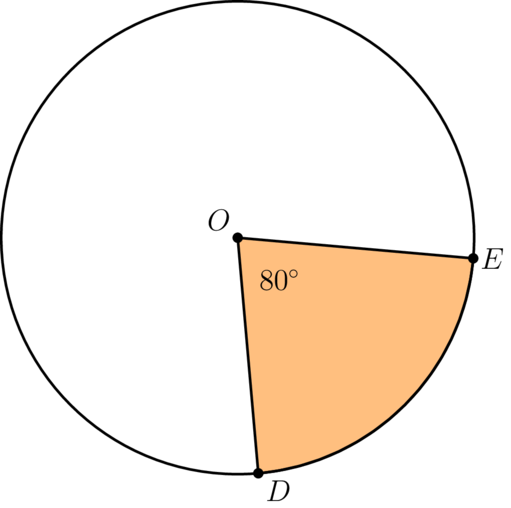Calculate what fraction of the area of the circle is shaded:

So, the shaded region is $\frac{2}{9}$ of the area of the whole circle.

Area of whole circle:

Diameter = 70 cm, so radius = 35 cm

\begin{align} A_{\text{whole circle}} & = \pi r^{2} \\ & = 3.14 \times 35^{2} \\ & = 3.14 \times 1,225\\ & = \text{3,846.5 mm}^{2}\\ \end{align}

\begin{align} A_{\text{sector}} & = A_{\text{whole circle}} \times \frac{2}{9} \\ & = 3,846.5 \times \frac{2}{9} \\ & = \text{854.777...mm}^{2}\\ & \approx \text{854.78 mm}^{2}\\ \end{align}

## 7.4. Practical applications

We often need to know how to calculate the areas of different shapes, for example, when we plan a new house or a vegetable patch in the garden.

Quite often we have to calculate the difference in area between two shapes, for example a circle and a triangle.

### Worked example 7.9: Calculating area of complex shapes

The following diagram shows a circle and an isosceles triangle drawn within the circle. The vertices of the triangle lie on the circumference of the circle. There is a dot at the centre of the circle. The area outside the triangle is shaded.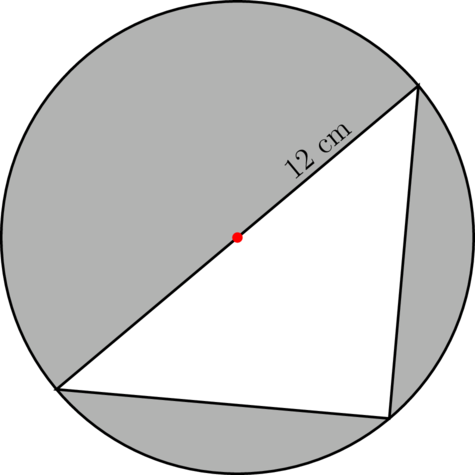Given that the radius of the circle is 12 cm, calculate the shaded area.

1. Step 1: Choose a strategy to carry out the calculation.

We need to calculate the area that has been shaded. To do that, we will calculate the areas of the two shapes separately, and then subtract the area of the triangle from the area of the circle.

2. Step 2: Calculate the area of the circle.

The area of the circle is:

\begin{align} A_{\text{circle}} & = \pi r^{2} \\ & = 3.14 \times 12^{2} \\ & = 3.14 \times 144 \\ & = \text{452.16 cm}^{2} \\ \end{align}
3. Step 3: Determine the base and the height of the triangle.

We know that the radius of the circle is 12 cm. The red dot is the centre of the circle, so any distance from that dot to the circle's circumference is 12 cm. This means we can label the diagram with the information as follows: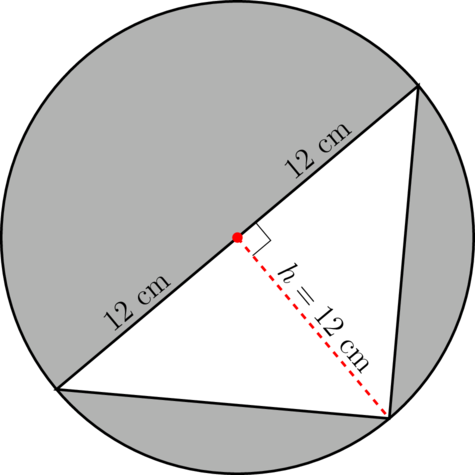The base of the triangle is therefore 12 $\times$ 2 = 24 cm.

The height is perpendicular to the base of the triangle, and is also a radius of the circle.

Height of the triangle = radius of the circle = 12 cm.

4. Step 4: Calculate the area of the triangle.

\begin{align} A_{\text{triangle}} & = \frac{1}{2}bh \\ & = \frac{1}{2} \times 24 \times 12 \\ & = \text{144 cm}^{2} \\ \end{align}
5. Step 5: Calculate the shaded area.

To find the shaded area, we need subtract the area of the triangle from that of the circle:

\begin{align} A_{\text{shaded area} } & = A_{\text{circle}} - A_{\text{triangle}} \\ & = 452.16 - 144 \\ & = \text{308.16 cm}^{2} \\ \end{align}

### Exercise 7.8: Calculate area of complex shapes

1. The following diagram shows a circle and an isosceles triangle drawn within the circle. The vertices of the triangle lie on the circumference of the circle. There is a dot at the centre of the circle. The area outside the triangle is shaded.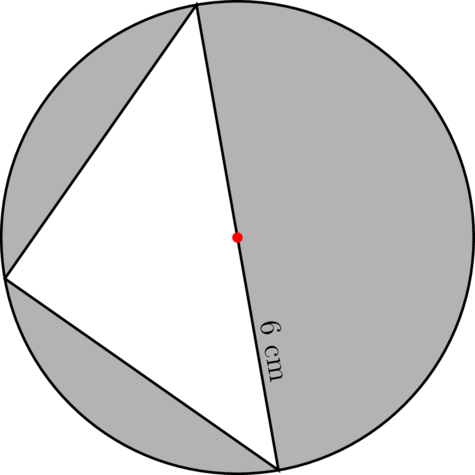Given that the radius of the circle is 6 cm, calculate the shaded area.

We need to calculate the areas of the two shapes separately, and then subtract the area of the triangle from the area of the circle.

Area of the circle:

\begin{align} A_{\text{circle}} & = \pi r^{2} \\ & = 3.14 \times 6^{2} \\ & = 3.14 \times 36 \\ & = \text{113.04 cm}^{2} \\ \end{align}

Base and height of the triangle:

The red dot is the centre of the circle, so any distance from that dot to the circle's circumference is 6 cm. We can label the diagram as follows: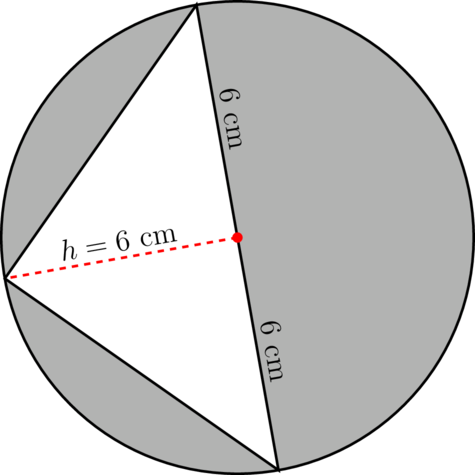The radius of the circle is 6 cm, so the diameter of the circle = 2 $\times$ 6 cm = 12 cm.

Therefore, the base of the triangle is 12 cm.

The height of the triangle = 6 cm.

Area of the triangle:

\begin{align} A_{\text{triangle}} & = \frac{1}{2}bh \\ & = \frac{1}{2} \times 12 \times 6 \\ & = \text{36 cm}^{2} \\ \end{align}

\begin{align} A_{\text{shaded area}} & = A_{\text{circle}} - A_{\text{triangle}} \\ & = 113.04 - 36 \\ & = \text{77.04 cm}^{2} \\ \end{align}
2. Mr and Mrs Chukwu bought a new property. There is a house, a vegetable garden and a lawn on the property. The diagram below shows the property.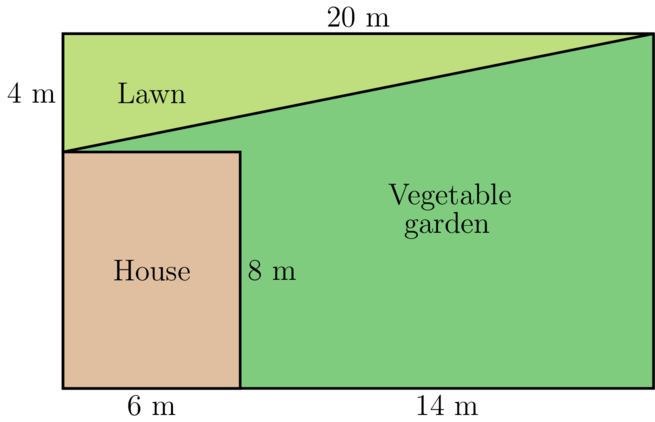1. Calculate the area of the property, in metres squared.
\begin{align} A_{\text{rectangle}} &= l \times b \\ &= 20 \times (4 + 8) \\ & = 20 \times 12 \\ & = \text{240 m}^{2} \\\ \end{align}
1. Calculate the area of the lawn.

The lawn is in the shape of a triangle.

\begin{align} A_{\text{triangle}} &= \frac{1}{2} \times b \times h \\ & = \frac{1}{2} \times 20 \times 4 \\ & = \text{40 m}^{2}\\ \end{align}
1. Calculate the area of the vegetable garden.

Strategy: Area of vegetable garden = Area of property $-$ area of house $-$ area of lawn

Area of house:

\begin{align} A_{\text{rectangle}} &= l \times b \\ &= 8 \times 6 \\ & = \text{48 m}^{2}\\ \end{align}

Area of vegetable garden:

\begin{align} A_{\text{vegetable garden}} & = A_{\text{property}} - A_{\text{house}} - A_{\text{lawn}} \\ & = 240 - 48 - 40\\ & = \text{152 m}^{2}\\ \end{align}
3. Mrs Jaja plans to build a cement dam for water next to her house. She has not decided on a shape for the new dam. Mrs Jaja has to choose between two options.

1. Option A is for Mrs Jaja to build a circular dam with a radius of 1.5 m, as shown in the diagram: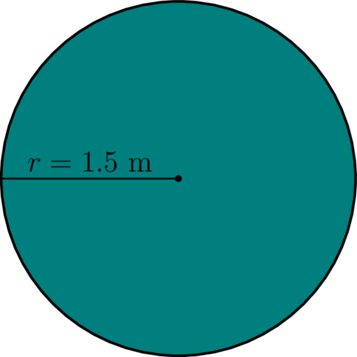Calculate the area of this dam in metres squared.

\begin{array}{ll} A_{\text{circle}} & = \pi r^{2} \\ & = 3.14 \times 1.5^{2} \\ & = 3.14 \times 2.25 \\ & = \text{7.065 m}^{2} \\ & \approx \text{7.07 m}^{2} \\ \end{array}
1. Option B is a suggestion by Mrs Jaja's neighbour. The neighbour designed a dam as shown below: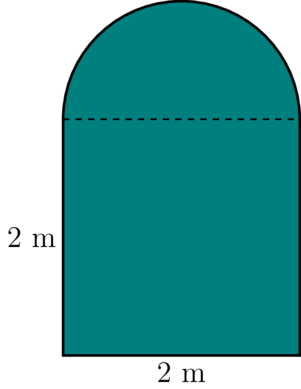Calculate the area of this dam. Use 3.14 for $\pi$ and round the answer off to two decimal places if necessary.

The dam consists of a square and one semi-circle.

Area of square:

\begin{align} A_{\text{square}} &= s \times s \\ &= 2 \times 2\\ & = \text{4 m}^{2}\\ \end{align}

Area of whole circle:

Diameter of circle = 2 m, so radius of circle = 1 m

\begin{align} A_{\text{circle}} & = \pi r^{2} \\ & = 3.14 \times 1^{2} \\ & = 3.14 \times 1 \\ & = \text{3.14 m}^{2} \\ \end{align}

Area of semi-circle:

\begin{align} A_{\text{semi-circle}} & = A_{\text{whole circle}} \times \frac{1}{2} \\ & = 3.14 \times \frac{1}{2} \\ & = \text{1.57 m}^{2}\\ \end{align}

Total area:

\begin{align} A _{\text{dam}} & = A_{square} + A_{semi-circle} \\ & = 4 + 1.57 \\ & = \text{5.57 m}^{2} \\ \end{align}
1. Which option should Mrs Jaja choose? Give a reason for your answer.

The area of the the Option B design is smaller than the area of the Option A design. If Mrs Jaja is concerned that the dam will take up too much space on the property, she should decide on Option B. The dam can have higher walls to hold enough water.

4. Mr Okoro wants to put paving around his new, triangular flower garden, as shown in the diagram.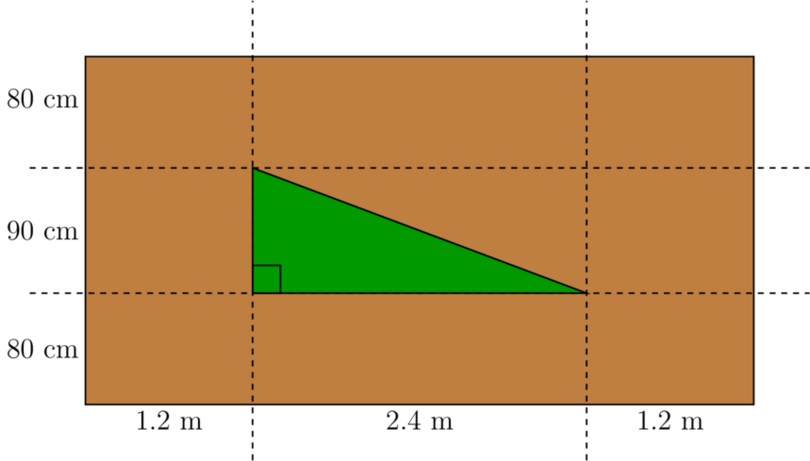1. What is the area of the flower garden in metres squared?

Area of flower garden (triangle):

• Base of triangle = 2.4 metres
• Height of triangle = 90 cm = 0.9 metres
\begin{align} A_{\text{triangle}} & = \frac{1}{2} \times b \times h\\ & = \frac{1}{2} \times 2.4 \times 0.9 \\ & = \text{1.08 m}^{2} \\ \end{align}
1. What is the area of the paving, in metres squared (excluding the flower garden)?

To find the area of the paving, we have to subtract the area of the flower garden from the total area.

Total area (rectangle):

• Length of rectangle = 1.2 + 2.4 + 1.2 = 4.8 metres
• Breadth of rectangle = 80 + 90 + 80 = 250 cm = 2.5 metres
\begin{align} A_{\text{rectangle}} & = l \times b\\ & = 4.8 \times 2.5 \\ & = \text{12 m}^{2} \\ \end{align}

Area of paving:

\begin{align} A_{\text{paving}} & = A_{\text{rectangle}} - A_{\text{triangle}} \\ & = 12 - 1.08 \\ & = \text{10.92 m}^{2} \\ \end{align}
5. Study the figure $OPQRS$ below. Measurements are given in centimetres.Calculate the area of the figure, using two different methods. Round your answer to one decimal place.

Method 1: Divide the figure into a rectangle and a triangle.

Area of rectangle:

\begin{align} A_{\text{rectangle}} & = \text{length} \times \text{breadth} \\ & = 10 \times 7 \\ & = \text{70 cm}^{2} \\ \end{align}

Area of triangle:

\begin{align} A_{\text{triangle}} & = \frac{1}{2} \times \text{base} \times \text{height} \\ & = \frac{1}{2} \times 10 \times 5 \\ & = \text{25 cm}^{2} \\ \end{align}

Total area:

\begin{align} A_{\text{total}} & = A_\text{rectangle} + A_\text{triangle} \\ &= 70 + 25 \\ & = \text{95 cm}^{2} \\ \end{align}

Method 2: Consider the shape to be a trapezium.

• Height of trapezium = $PQ$ = 10 cm
• $a$ = $OS$ = 7 cm
• $b$ = $PQ$ + $QR$ = 7 + 5 = 12 cm

Area of trapezium:

\begin{align} A_{\text{trapezium}} &= \frac{h}{2}\left( a + b \right) \\ & = \frac{10 }{2}\left( 12 + 7 \right)\\ & = 5 \times \left(19 \right)\\ & = \text{95 cm}^{2}\\ \end{align}

Conclusion: The two methods give the same answer.

6. The diagram below shows a circular dam surrounded by a path. The diameter of the dam is 10 m, and the path is 3 m wide all the way around.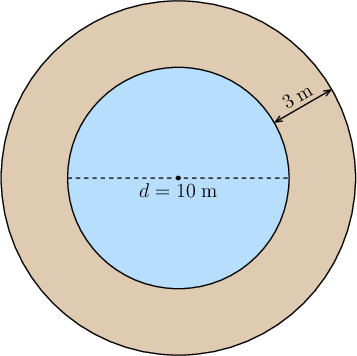Calculate the area of the path.

Strategy: The area of the path is the difference in area between the two circles. To calculate the area of the path, we must subtract the area of the small circle from the area of the big circle.

Let the radius of the small circle be equal to $r$.

\begin{align} r & = d \div 2\\ & = 10 \div 2 \\ & = \text{5 m}\\ \end{align}

Area of small circle:

\begin{align} A_{\text{small circle}} & = \pi r^{2} \\ & = 3.14 \times 5^{2} \\ & = 3.14 \times 25 \\ & = \text{78.5 m}^{2} \\ \end{align}

Let the radius of the big circle be equal to $R$.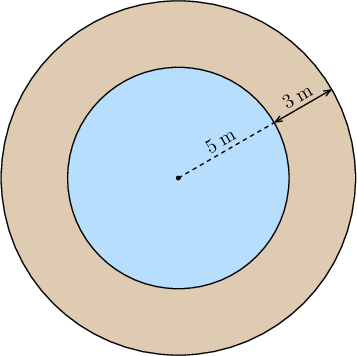\begin{align} R & = r + 3 \\ & = 5 + 3 \\ & = \text{8 m}\\ \end{align}

Area of big circle:

\begin{align} A_{\text{big circle}} & = \pi R^{2} \\ & = 3.14 \times 8^{2} \\ & = 3.14 \times 64 \\ & = \text{200.96 m}^{2} \\ \end{align}

Area of path:

\begin{align} A_{\text{path}} & = A_{\text{big circle}} - A_{\text{small circle}} \\ & = 200.96 - 78.5 \\ & = \text{122.46 m}^{2} \\ \end{align}
7. Ms Ali works for a company that lays paving around swimming pools. She must calculate the area that must be paved for the swimming pool shown below.

Calculate the total area of the pool and calculate the area that must be paved.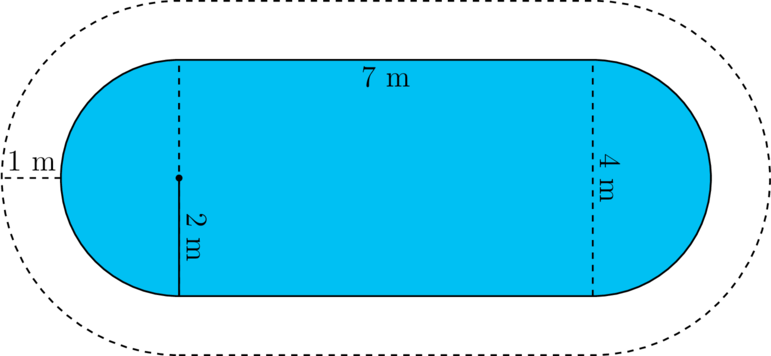Area of the pool:

The pool consists of a rectangle and two semi-circles.

The two semi-circles form one whole circle.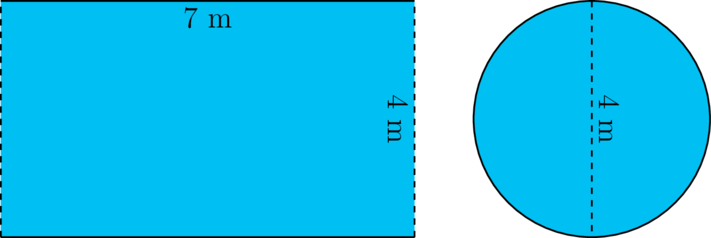Area of rectangle:

\begin{align} A_{\text{rectangle}} & = l \times b\\ & = 7 \times 4\\ & = \text{28 m}^{2} \\ \end{align}

Area of circle:

\begin{align} A_{\text{circle}} & = \pi r^{2} \\ & = 3.14 \times 2^{2} \\ & = 3.14 \times 4 \\ & = \text{12.56 m}^{2} \\ \end{align}

Total area of swimming pool:

\begin{align} A_{\text{swimming pool} } & = A_{\text{rectangle}} + A_{\text{circle}} \\ & = 28 + 12.56 \\ & = \text{40.56 m}^{2} \\ \end{align}

Area of paving next to rectangle:

The paving next to the rectangle consists of two rectangles. Each of these rectangles has dimensions 7 m by 1 m.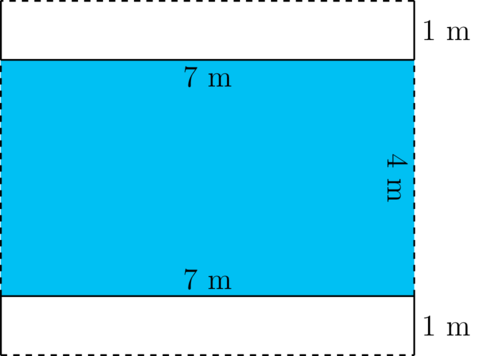\begin{align} A_{\text{two rectangles}} & = 2 \times l \times b\\ & = 2 \times 7 \times 1\\ & = \text{14 m}^{2}\\ \end{align}

Area of paving next to semi-circles:

To calculate the paving next to the two semi-circles, you have to calculate the total circular area (area of pool and paving) first, and then subtract the circular area of the pool.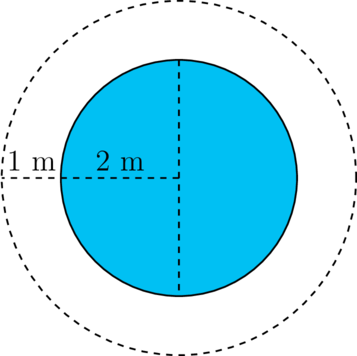Total area (pool and paving) of circle:

Let radius of total circular area = $R$

\begin{align} R & = 2 + 1 \\ & = \text{3 m} \\ \end{align} \begin{align} A_{\text{circle}} & = \pi R^{2} \\ & = 3.14 \times 3^{2} \\ & = 3.14 \times 9 \\ & = \text{28.26 m}^{2} \\ \end{align}

Area of paving around two semi-circles:

We already calculated the area of the circular part of the pool above: 12.56 m $^{2}$

\begin{align} A_{\text{paving}} & = A_{\text{total}} - A_{\text{pool}} \\ & = 28.26 - 12.56 \\ & = \text{15.7 m}^{2} \\ \end{align}

Total paving:

\begin{align} A_{\text{total}} & = A_{\text{paving next to rectangle}} + A_{\text{paving next to semi-circles}} \\ & = 14 + 15.7\\ & = \text{29.7 m}^{2} \\ \end{align}

## 7.5 Summary

• Area is the amount of flat space taken up by a shape.
• A plane shape is a closed shape on a flat surface, for example a triangle, parallelogram, trapezium or circle. It is also called a two-dimensional shape.
• Rectangle:
• The area of the rectangle is: $A = \text{base} \times \text{height}$.
• Triangle:
• The area of the triangle is half of the area of a rectangle.
• The formula for the area of a triangle is $A_{\text{triangle}} = \frac{1}{2} \times b \times h$.
• The height of a triangle must be perpendicular to the base of the triangle.
• The height of a triangle can be inside or outside the triangle.
• In a right-angled triangle, either of the two sides that meet at the right angle can be the height of the triangle.
• Parallelogram:
• A parallelogram is a quadrilateral with two pairs of opposite sides that are equal and parallel.
• The formula for the area of a parallelogram is $A_{\text{parallelogram}} = b \times h$.
• The height ( $h$) must be perpendicular to the base ( $b$).
• Trapezium:
• A trapezium is a quadrilateral with one pair of opposite sides that are parallel.
• The formula for the area of a trapezium is $A_{\text{trapezium}} = \frac{h}{2}\left( a + b \right)$.
• The two parallel sides of the trapezium ( $a$ and $b$) must be perpendicular to the height ( $h$) of the trapezium.
• Circle:
• A diameter of a circle is a line segment that joins two points on the circumference of the circle and passes through the centre of the circle.
• The diameter of a circle is double the length of the radius of the same circle.
• A radius of a circle is a line segment that joins the centre of the circle to a point on the circumference of the circle.
• A sector of a circle is the area enclosed between two radii and the circumference of the circle.
• The formula for the area of a circle is ${A_{\text{circle}} = \pi r^{2}}$.
• A semi-circle is a half of a full circle, so its area formula is $\text{Area of semi-circle}$ = $\text{area of whole circle } \times \frac{1}{2}$.
• A quarter-circle is a quarter of a full circle, so its area formula is $\text{Area of quarter circle}$ = $\text{area of whole circle } \times \frac{1}{4}$.
• We can calculate what fraction a sector is of a circle using $\frac{\text{angle of sector}}{360^{\circ}}$.
• The formula for the area of a sector is $\text{Area of circle sector}$ = $\text{area of whole circle } \times \text{fraction of circle that is shaded}$.
• Summary of area formulae:
Shape Area formula
Rectangle $A = bh$
Triangle $A = \frac{1}{2}bh$
Parallelogram $A = bh$
Trapezium $A = \frac{h}{2}\left( a + b \right)$
Circle $A = \pi r^{2}$
• We use our knowledge of formulas of areas in practical applications.